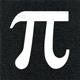# Math Is Fun Forum

Discussion about math, puzzles, games and fun.   Useful symbols: ÷ × ½ √ ∞ ≠ ≤ ≥ ≈ ⇒ ± ∈ Δ θ ∴ ∑ ∫  π  -¹ ² ³ °

You are not logged in.

## #1 2017-01-15 10:02:35

zetafunc
ModeratorRegistered: 2014-05-21
Posts: 2,386
Website

Suppose that we have a sequence of
brackets, which must obey the usual open/close law -- that is, sequences like (()), ()()(), (())(()) are allowed, but not sequences like ))(( or )()(. Moreover, the number of left brackets must be equal to the number of right brackets.

Now, starting from left to right, suppose we take some subset of the first
brackets, where
Let
denote the number of left brackets, and
denote the number of right brackets in this subset. For example, if we consider the sequence ()()()(), then for how many values of
do we have
?

For example, suppose I take the sequence ()()(). Then there are three values of
for which
, namely,
If instead we took the sequence (()) then there is only one value --

How can we use a generating function, or other combinatorial argument to enumerate all the possibilities? If the order didn't matter, then we could find the coefficient of
in the expansion of
, which is
But this includes those combinations such as )()(, which isn't allowed. How can we change this to account for the constraints above?

I suspect that the answer should be

Last edited by zetafunc (2017-01-15 11:20:56)

Offline

## #2 2017-01-15 12:07:57

bobbym
bumpkinFrom: Bumpkinland
Registered: 2009-04-12
Posts: 109,606

### Re: Combinatorics question about brackets

Hi;

I do not understand about R(t), L(t), can you explain further.

The numbers you are suggesting are every other Catalan number.

In mathematics, you don't understand things. You just get used to them.
If it ain't broke, fix it until it is.
Always satisfy the Prime Directive of getting the right answer above all else.

Offline

## #3 2017-01-15 12:15:57

zetafunc
ModeratorRegistered: 2014-05-21
Posts: 2,386
Website

### Re: Combinatorics question about brackets

Suppose we have a sequence (()()) -- that's 6 brackets long. Then:

L(1) = 1, R(1) = 0 -- taking the first bracket
L(2) = 2, R(2) = 0 -- taking the first two brackets
L(3) = 2, R(3) = 1 -- taking the first three brackets
L(4) = 3, R(4) = 1 -- taking the first four brackets
L(5) = 3, R(5) = 2 -- taking the first five brackets
L(6) = 3, R(6) = 3 -- taking all six brackets

In this case, we see that the only value of t for which L(t) = R(t) is t = 6.

Yes, they are the Catalan numbers. But I'd like to know how to get that result combinatorially.

Last edited by zetafunc (2017-01-15 12:19:45)

Offline

## #4 2017-01-15 12:40:23

bobbym
bumpkinFrom: Bumpkinland
Registered: 2009-04-12
Posts: 109,606

### Re: Combinatorics question about brackets

Hi;

I think I understand the problem now. I have notes on this bracketing problem somewhere. I am looking for them now.

In mathematics, you don't understand things. You just get used to them.
If it ain't broke, fix it until it is.
Always satisfy the Prime Directive of getting the right answer above all else.

Offline

## #5 2017-01-15 12:54:58

zetafunc
ModeratorRegistered: 2014-05-21
Posts: 2,386
Website

### Re: Combinatorics question about brackets

OK, let me know what you find.

I found this: http://www-math.mit.edu/~djk/18.310/18. … theses.pdf

Offline

## #6 2017-01-15 12:59:03

bobbym
bumpkinFrom: Bumpkinland
Registered: 2009-04-12
Posts: 109,606

### Re: Combinatorics question about brackets

Hi;

Thanks for the link. I understand how you want to count them say for ()()(). But what is the question you are asking?

I do see the formula you are conjecturing on page 5 of that pdf.

In mathematics, you don't understand things. You just get used to them.
If it ain't broke, fix it until it is.
Always satisfy the Prime Directive of getting the right answer above all else.

Offline